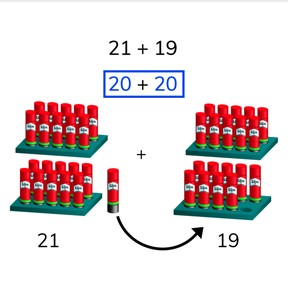Addition to 100 with round numbers

# Addition to 100 with round numbers8,000 schools use Gynzy92,000 teachers use Gynzy1,600,000 students use Gynzy

## General

Students learn adding to 100 by using round numbers. They compensate within the addition problem to create round numbers.

2.NBT.B.5

## Relevance

It is important to learn how to do this to make adding to 100 easier and faster.

## Introduction

Ask students to find their way out of the addition maze. The first addend is given, and students must add to total 100. They must find their way out of the maze.

## Development

Explain to students how they can add to 100 easily using compensation within the problem. If you can make both addends round by taking something away from one and adding it to the other it is adding with round numbers using compensation within the problem.
You can solve a problem like 29+31 using this. You make the addends round. You do this by taking 1 from 31 and adding that one to 29 to make 30. That means the problem you solve is 30 + 30 = 60. Remind the students that this total is the same as solving 29+31, but is easier to do. Using the problem 42+48 how to apply the strategy the other way around. You take 2 from the first addend and add them to the second addend. That makes 40+50. Again, the total remains the same. Ask students to practice with an exercise without visual support.

Check that students are able to add using round numbers by asking the following questions:
- How do you solve 17+33 making round numbers? (+3/-3)
- Why is it useful to add by rounding the numbers? (you are able to solve easier)

## Guided practice

Students first practice solving an addition problem with visual support, rounding both addends. They then practice doing so without visual support.

## Closing

The interactive whiteboard shows 9 random numbers below 100. Ask the students to round the numbers by adding something or taking something away. 17 becomes 20, 58 becomes 60 and so on. Discuss why it is useful to round your addends before adding them together. Point out that what you add to one addend must be taken from the other addend to make sure your answer stays correct.

## Teaching tips

For students who have difficulty with rounding the addends, it can be useful for them to use MAB-blocks or other manipulatives to help visualize the process. They can then clearly see that what they take from one group they add to another group, but that the total remains the same. For students who are looking to be challenged, encourage them to solve these problems in their heads.

## Instruction materials

Optional: MAB-blocks or other manipulatives

### The online teaching platform for interactive whiteboards and displays in schools

• Save time building lessons

• Manage the classroom more efficiently

• Increase student engagement